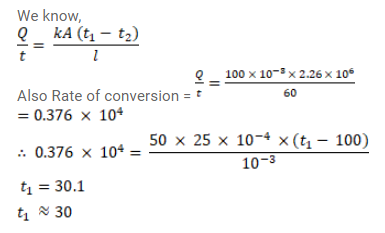# Water is boiled in a container having a bottom of surface areaQuestion:

Water is boiled in a container having a bottom of surface area $225 \mathrm{~cm}^{2}$, thickness $1.0 \mathrm{~mm}$ and thermal conductivity $50 \mathrm{~W} / \mathrm{m}-{ }^{\circ} \mathrm{C} 100 \mathrm{~g}$ of water is converted into steam per minute in the steady state after the boiling starts. Assuming that no heat is lost to the atmosphere, calculate the temperature of the lower surface of the bottom. Latent heat of vaporization of water $=2.26 \times 10^{6} \mathrm{~J} / \mathrm{kg}$.

Solution: